International Tables for Crystallography (2006). Vol. A, ch. 8.3, pp. 732-740https://doi.org/10.1107/97809553602060000516

## Contents

• 8.3. Special topics on space groups  (pp. 732-740)
• 8.3.1. Coordinate systems in crystallography  (p. 732) | html | pdf |
• 8.3.2. (Wyckoff) positions, site symmetries and crystallographic orbits  (pp. 732-734) | html | pdf |
• 8.3.3. Subgroups and supergroups of space groups  (pp. 734-736) | html | pdf |
• 8.3.3.1. Translationengleiche or t subgroups of a space group  (p. 735) | html | pdf |
• 8.3.3.2. Klassengleiche or k subgroups of a space group  (p. 735) | html | pdf |
• 8.3.3.3. Supergroups  (pp. 735-736) | html | pdf |
• 8.3.4. Sequence of space-group types  (p. 736) | html | pdf |
• 8.3.5. Space-group generators  (pp. 736-738) | html | pdf |
• 8.3.5.1. Selected order for non-translational generators  (pp. 737-738) | html | pdf |
• 8.3.6. Normalizers of space groups  (pp. 738-739) | html | pdf |
• References | html | pdf |
• Figures
• Fig. 8.3.3.1. Space groupwith t subgroups of index  and   (p. 735) | html | pdf |
• Fig. 8.3.6.1. Square with `symmetry elements'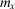,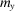,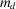,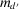(mirror lines) and ♦ (fourfold rotation point)  (p. 739) | html | pdf |
• Tables
• Table 8.3.4.1. Listing of space-group types according to their geometric and arithmetic crystal classes  (p. 736) | html | pdf |
• Table 8.3.5.1. Sequence of generators for the crystal classes  (p. 737) | html | pdf |
• Table 8.3.5.2. Example of a space-group generation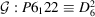(No. 178)  (p. 738) | html | pdf |